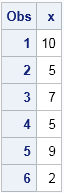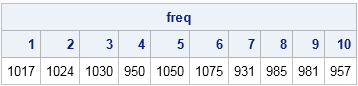You can use SAS to generate random integers between 1–10 or in the range 1–100. This article shows how to generate random integers as easily as Excel does.

I was recently talking with some SAS customers and I was asked "Why can't SAS create an easy way to generate random numbers? Excel has a simple way to generate random numbers between 1 and 100, and I use it all the time."

The speaker was talking about generating random integers from a discrete uniform distribution, where the numbers range between a specified minimum and maximum value. I have previously written about how to generate random numbers in SAS, but the section about random integers is buried in the middle. So let's simplify the process and see whether we can get SAS to generate random integers "as easily as Excel does."

### Built-in support for random integers

If you have access to SAS 9.4M5, you can generate random integers directly. The RAND function supports the "Integer" distribution and supports parameters that specify the min and max. To generate a random integer between 1 and 10 you can use the following statements:

```data RandInt; do i = 1 to 100; x = rand("Integer", 1, 10); /* requires SAS 9.4M5 or later */ output; end; run;```

### A macro with a simple syntax

For older versions of SAS, you can define a macro. I looked up the Excel function that generates random integers. The syntax is RANDBETWEEN(min, max). For example, if you want a random integer between 1 and 10, the syntax is RANDBETWEEN(1, 10). For a random integer between 1 and 100, use RANDBETWEEN(1, 100).

The following SAS macro hides the complexity of the RAND function and creates a simple statement that that has the same functionality as the Excel function. It generates random uniform numbers in (0, 1), then transforms them to integers in [min, max]:

```/* SAS macro that duplicates the Excel RANDBETWEEN function */ %macro RandBetween(min, max); (&min + floor((1+&max-&min)*rand("uniform"))) %mend;```

After you've defined the macro, you can just pretend that it is a function and use it in a DATA step. The following DATA step generates 100 random numbers between 1 and 10:

```data RandInt; do i = 1 to 100; x = %RandBetween(1, 10); output; end; run;   proc print data=RandInt(obs=6); var x; run;```The adjacent table shows the first six random integers. Every time you run the program you will get a different sequence of random integers. Use the STREAMINIT subroutine if you want a reproducible stream of integers.

### A SAS/IML function for random integers

The previous sections are for DATA step programmers. SAS/IML 14.2 also supports the "Integer" distribution. For earlier versions of SAS/IML, you can define a function that takes an additional argument and returns a vector of random integers:

```proc iml; /* Generate n random integers drawn uniformly at random from integers in [min, max] where min and max are integers. */ start RandBetween(n, min, max); u = j(n, 1); call randgen(u, "Uniform", min, max+1); return( floor(u) ); finish;   /* Test the function. Generate 10,000 values and tabulate the result. */ call randseed(1234); x = RandBetween(10000, 1, 10); call tabulate(val, freq, x); print freq[c=(char(val))];```The output shows that each integer 1 through 10 appeared approximately 1,000 times in the simulated data.

In summary, modern versions of SAS support the "Integer" distribution for generating random integers. For older versions of SAS, you can define a SAS macro that hides the complexity of the RAND function. The result is a syntax that is easy to use and duplicates the functionality of the Excel RANDBETWEEN function.

ShareDistinguished Researcher in Computational Statistics

Rick Wicklin, PhD, is a distinguished researcher in computational statistics at SAS and is a principal developer of SAS/IML software. His areas of expertise include computational statistics, simulation, statistical graphics, and modern methods in statistical data analysis. Rick is author of the books Statistical Programming with SAS/IML Software and Simulating Data with SAS.

1.Hi Rick

Thanks for this example. With Proc FCMP we can also create our own functions in Base SAS. Example below:

proc fcmp outlib=work.myfunc.sample;
function RandBetween(min, max);
return (min + floor((1 + max - min) * rand("uniform")));
endsub;
run;

options cmplib=work.myfunc;

data RandInt;
do i = 1 to 100;
x2 = RandBetween(101, 110);
output;
end;
run;

•Except that user-written functions aren't usable within SQL. But this works just fine:

proc sql; create table foon as
SELECT %RandBetween(10**12, (10**13)-1) as claim,...

•I can use my functions in Proc SQL? Am I missing something?

2.That looks like an excellent (or non-Excel-ent) way to solve the problem!

;-)

•I'm a fan of excel and SAS
i'd never need that rand in excel but often use int(ranuni(0) * 100)
So for a team of analysts moved over to SAS probably write macro parameters like:
Choice of range, distribution and initial seed.
(sometimes it is good to have predictable random results and at others I wanted to check stability of a model)

3.Stephen Dorneman on

Love this macro, great work, thanks.

Any thoughts on how to extend it for non-integer data, with non-integer Max and Min values?

Thanks,
Stephen

•Yes. Click on the link in the second paragraph.

•Stephen Dorneman on

Thanks!

4.Hey Rick,
thank you so much for this example, really helpful..
This may be a bit off-topic here but may I ask you if you have any idea how to generate
correlated "random" data in sas? I am a beginner with the program and could not really
figure out how to do this.

Thank you so much, Julia

•Yes, I dedicate several chapters to that topic in my book Simulating Data with SAS. Since you did not specify the distribution, I will guess that you might mean correlated multivariate normal data. See "Sampling from the multivariate normal distribution," or if you don't have SAS/IML software use the SIMNORMAL procedure in SAS/STAT software.

•thank you so much for this. actually i want to show the adverse effects of cluster sampling when the data in respective clusters is correlated.
for this i want to generate a population with different clusters and ideally pre-define rho in the cluster.
i am a bit stuck there so really sorry if this question appears too basic. thanks anyways for your patience. j

•It sounds like you want a mixture of normal distributions. You can post questions like this to the SAS Support Communities. There is a community for SAS/IML software (best for multivariate simulations, if you know matrix programming) and another community for statistical procedures.

5.Thanks, Rick! This is great! Do you also have a blog on how to generate x random numbers from a (eg) normal distribution, given the mean, SD, and min/max?
I'm having trouble combining the instructions from both blogs to arrive at a combined program.

•If you want a random sample from a normal distribution, you can use the following:

```%let N = 50; /* sample size */ %let mu = 5; /* population mean */ %let sigma = 3; /* population SD */ data Normal(keep=x); call streaminit(12345); /* set random number seed */ do i = 1 to &N; x = rand("Normal", &mu, &sigma); output; end; run;```
•Thank you, Rick! How do I incorporate "max" and "min" into this?

•I don't understand your question. You can ask questions, post programs and data, and get advice from hundreds of experts at the SAS Support Communities.

•I have the same question -- how to create a NORMAL distribution within a specified range (min and max)? Not a uniform distribution.

•Technically, I don't really need a specified mean and SD -- I just need to generate a normal distribution that would fit within a specified range. I realize though that a normal distribution is by definition infinite... Are there any alternatives? E.g., a distribution that will have a shape and properties similar to those of a normal distribution but for which I can restrict range?

Thank you.

•You have a few options. Although the normal distribution is unbounded, the probability is only 1 / 3.5 million that a random value will be outside of the range mu +/- 5*sigma, where sigma is the standard deviation. So if you want normal data in [a,b], you can simulate from N( (a+b)/2, (b-a)/10 ). If you want a distribution that truly has finite support, you can scale and translate the Beta(a,a) distribution with a much greater than 1. For example, Beta(5,5) is symmetric and "bell shaped" on the interval (0,1). Scale and translate to get any other interval.

6.Thanks Rick!

New SAS user here. Your RandBetween Macro saved me a TON of time. Awesome logic!

•So glad to hear. Thanks for writing!

7.Thanks again Rick ... I'm generating some customer satisfaction ratings data and this macro really does the trick.

•Great! Thank YOU for the suggestion.

And more good news: in SAS 9.4M5 you don't need the macro anymore. The RAND function now supports the "Integer" distribution and supports min and max as parameters. To generate a random integer between 1 and 10 you can now say

`x = rand("Integer", 1, 10); /* random integer between 1 and 10 */`
8.That is useful, thanks! But have to hassle SI about sneaking it in: I have 2 'fifth editions' of the Functions Reference (PDF), and one documents it and the other doesn't — I normally read about new features in the What's New of new editions.

9.Ted Russell on

Is there any way of using Randint to make a non-duplciated list of random numbers?

Thanks

•I think you are asking for "sampling without replacement," whereas the topic of this article is sampling with replacement. If you want to use all numbers within a list, then sampling without replacement is a random permutation of the integers. You can obtain a random permutation in the DATA step by using the RANPERM function:

```data Want; seed = 12345; array x (1 2 3 4 5 6 7 8 9 10); call ranperm(seed, of x[*]); run; proc print; run;```

•Ted Russell on

Super, thanks for the speedy reply

10.Using SAS 9.4 TS1M4 under Windows, the 'INTEGER' argument to the RAND function is declared invalid. Is this a known issue?

•Thanks for catching that. I thought it was 9.4M4, but based on your comment I must have misremembered. The "Integer" distribution is available in 9.4M5. I correct that sentence in the article.

11.Rick,

Thank you for yet another eminently practical, "make your life easier" blog post. Your blog is one of the first I turn to when I need ideas for SAS.

By the way, with a few quick mods, the macro can be extended such that it can be executed outside a Data step. This (for me) is useful for generating code for test cases. See code below.

Regards,

Jim

%MACRO Rand_Between(Min, Max, Macro=NO);
%IF %QUPCASE(&Macro) = YES %THEN
%DO;
%EVAL(&Min + %SYSFUNC(FLOOR( %SYSEVALF( %EVAL(1 + &Max - &Min) * %SYSFUNC(RAND(Uniform)) ) )))
%END;
%ELSE
%DO;
(&Min + FLOOR((1 + &Max - &Min) * RAND("Uniform")))
%END;
%MEND Rand_Between;

•Thanks, Jim. As I say in the second section, you don't even need the macro in modern versions of SAS. Just use rand("Integer", 1, 10); That should work from anywhere that you can call Base SAS functions (FCMP, WHERE clauses, etc)

12.I want to randomize numbers (10180, 49740) can you help how to go about it. I tried the following :

%macro Randbetween(10180, 49740);
(&min + floor((1+-&min)*rand("uniform")))
%mend;
do i=1 to 100;
Cbsa_codeR = %RANDBetween(10180, 49740); (here didn't like the % in front of RANDBetween).
output;
end;

run;
proc print data=test(obs=200); var Cbsa_code; run; */;

•Don't change the definition of the RandBetween macro. Just call it with your parameters:

```%macro RandBetween(min, max); (&min + floor((1+&max-&min)*rand("uniform"))) %mend;   data Want; do i=1 to 100; Cbsa_codeR = %RANDBetween(10180, 49740); output; end; run;   proc print; run;```
13.To make it clear the lowest observation is 10,180 and the highest is 49, 740. THanks,